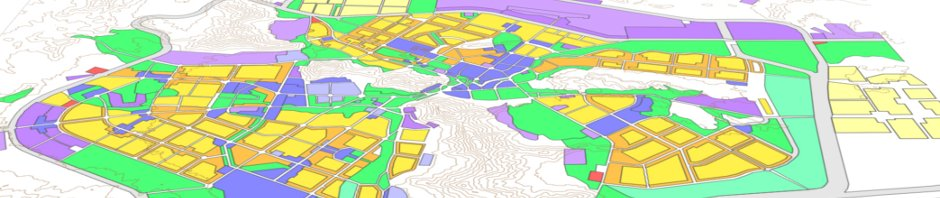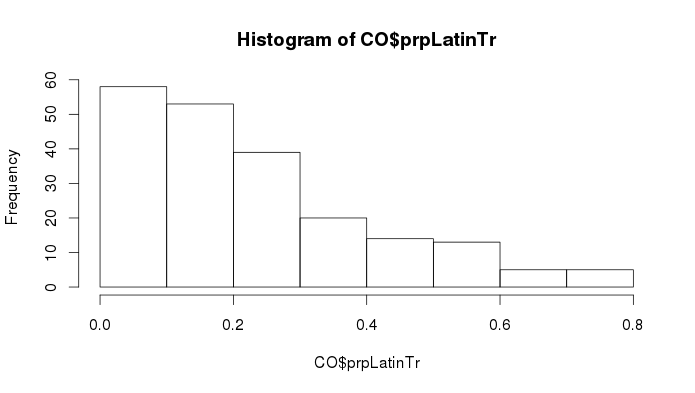# R: Chi-Squared Analysis

Regression analysis is a way to study the relationship between two variables with continuous data–what is called the “interval/ratio” Level of Measurement in research design.
Chi-squared analysis is designed to evaluate the relationship between two variables where the data is discrete, either in different conceptual categories (nominal Level of Measurement) or ranked-but-not-continuous data (Ordinal Level of Measurement).

With Census data, the particular variables may have continuous data, such as the population of African-Americans in Census Tracts in a county. But the distinction of races/ethnicities is a categorical distinction, so a comparison across multiple races means either running separate linear models (or a multiple regression), or it means comparing categorically discrete data all together. Furthermore, even though the data within categories is continuous, it can also be divided into groupings above and below fixed thresholds. For instance, you could distinguish dense Census Tracts from low-density/sprawled Census Tracts; you could distinguish relatively high concentrations of a population group from relatively low concentrations.

On this page I will show how to categorize continuous data in meaningful ways, and then how to evaluate relationships between categorically-discrete variables in R. The Chi-squared test will enable you to test basic statistical hypotheses about the relationship between variables like relative ethnic concentrations and relative population-density.

### X2 analysis, step 1: identify/create your discrete variables

To set thresholds for discrete high/low variables, it helps to look at the distribution of the data. Here I will create a histogram of the proportion of Latinos in Tracts:

```# View data-distribution of Latinos in county:
hist(CO\$prpLatinTr)```In this histogram, the proportion drops off significantly at the 0.3 threshold. In Contra Costa County, a Census Tract with over 30% Latin Americans is relatively rare. Note: relative concentrations in your county will vary significantly from this. Choose your group appropriate to your county, review your histogram, and set your high/low threshold accordingly.

Here is the plain-language description of what I will do: I will use 30% as the threshold measure to divide my Tract data into two conditions: HiLat and LoLat. I will use the ifelse() function to set a test-threshold and have it assign HiLat if true, and LoLat if false. Here is the R-syntax for this part of the operation (don’t run this yet):

```# partial formula for creating a new "threshold"-variable:
ifelse(CO\$prpLatinTr > 0.3, "HiLat", "LoLat")```

I will name this new variable hiloLatTr, and I will add this new variable as a column within the dataframe “CO”. Here is the R-syntax for the whole function:

```# Create threshold-variable of Hi/Lo Latino proportion, > 0.3
CO\$hiloLatTr = ifelse(CO\$prpLatinTr > 0.3, "HiLat", "LoLat")```

Refresh the view of your dataframe “CO”, and you should see a new column named “hiloLatTr”, and the data in that column should read either “HiLat” or “LoLat” all the way down.

I will do the same for relative density. First, a graphic review of the distribution:

```# View the density-distribution of the county:
hist(CO\$Dens_Tr)```

Set threshold at 5 people / acre, and create the new variable “hiloDnsTr” within dataframe “CO”:

```# Create threshold-variable of Hi/Lo pop density, > 5 ppl/ac
CO\$hiloDnsTr = ifelse(CO\$Dens_Tr > 5.0, "HiDens", "LoDens")```

### X2 analysis, step 2: create a Contingency Table of your variables:

So, are Tracts in CoCo with relatively higher concentrations of Latinos also more likely to be relatively dense? To find this out, I create a Contingency Table:

```# Create contingency table of density-by-Latinity:
Lat_Dns_tbl = table(CO\$hiloDnsTr,CO\$hiloLatTr)```

…and then view the table:

```# View the contingency table:
Lat_Dns_tbl```
```         HiLat LoLat
HiDens    34    50
LoDens    23   100```

Either way you read this table, it looks like higher proportion of Latinos is associated with a higher density. But what is the strength of this association?

### X2 analysis, step 3: run the chi-squared test

I have created a Contingency Table named “Lat_Dns_Tbl”. To run a chi-squared test, I use the chisq.test() command:

```# Run a Chi-squared test of probabilities in this table:
chisq.test(Lat_Dns_tbl)
# R returns this feedback:
Pearson's Chi-squared test with Yates' continuity correction
data:  Lat_Dns_tbl
X-squared = 10.7965, df = 1, p-value = 0.001017```

### X2 analysis, step 4: evaluate p-value for hypothesis-test

R not only runs the test, but it also looks up the p-value based on the degrees-of-freedom (df) in the test. In this case, the odds that there is no relationship between concentration of Latinos and population-density are about one in one thousand (0.001). In which case, the null-hypothesis (no relationship) can be rejected with a confidence of more than 99%; almost 99.9%.

That’s it. Now I want you to design and run your own chi-squared test on your own data by Tuesday, December 2.